# Introduction to Spatial data in R

We present some basic tools to work with spatial data in R. Some examples were taked form intert and I’ll put the orignal link as soon as I remember the source. Feel free to send the link if you know.

## Setup

### R and RStudio

To avoid problems, please start by updating your installation of R to the latest version. Installers for R can be found here https://cloud.r-project.org/

### Library installation

In this tutorial, we will work with three libraries:

• `sp`: for working with vector data,
• `rgdal`: for importing and exporting vector data from other programs,
• `raster`: for working with raster data,
• `tidyverse`: for data wrangling (optional).

On Windows:

Just run: `install.packages(c("sp", "raster", "rgdal"))`

On Mac:

If everything went well, the following three commands should run without problem!

``````    library(sp)
library(rgdal)``````
``````## rgdal: version: 1.3-9, (SVN revision 794)
##  Geospatial Data Abstraction Library extensions to R successfully loaded
##  Loaded GDAL runtime: GDAL 2.3.2, released 2018/09/21
##  Path to GDAL shared files: /usr/share/gdal
##  GDAL binary built with GEOS: TRUE
##  Loaded PROJ.4 runtime: Rel. 5.1.0, June 1st, 2018, [PJ_VERSION: 510]
##  Path to PROJ.4 shared files: (autodetected)
##  Linking to sp version: 1.3-1``````
``    library(raster)``
``````##
## Attaching package: 'raster'``````
``````## The following object is masked from 'package:dplyr':
##
##     select``````
``````## The following object is masked from 'package:tidyr':
##
##     extract``````

## Data Structures

### Spatial Data in R

Almost all spatial structures in R are based on the `sp` package. There are three main types of spatial data we’ll work with: points, lines, and polygons.

There are three basic steps to creating spatial data by hand:

• Create geometric objects (points, lines, or polygons)
• Convert those geometric objects to `Spatial*` objects (`*` stands for Points, Lines, or Polygons)

To make them spatial objects, we also need to include information on how those x-y coordinates relate the places in the real world using a Coordinate Reference System (CRS).

### First Spatial* Object: `SpatialPoints`

Points are the most basic geometric shape, so we begin by building a `SpatialPoints` object.

A points is defined by a pair of x-y coordiantes, so we can create a set of points by (a) creating matrix of x-y points, and (b) passing them to the `SpatialPoints` function to create our first `SpatialPoints` object.

``````coordinates <- rbind(c(1.5, 2.00),
c(2.5, 2.00),
c(0.5, 0.50),
c(1.0, 0.25),
c(1.5, 0.00),
c(2.0, 0.00),
c(2.5, 0.00),
c(3.0, 0.25),
c(3.5, 0.50)) ``````
``````spatial.points <- SpatialPoints(coordinates)
# ..converted into a spatial object
plot(spatial.points)``````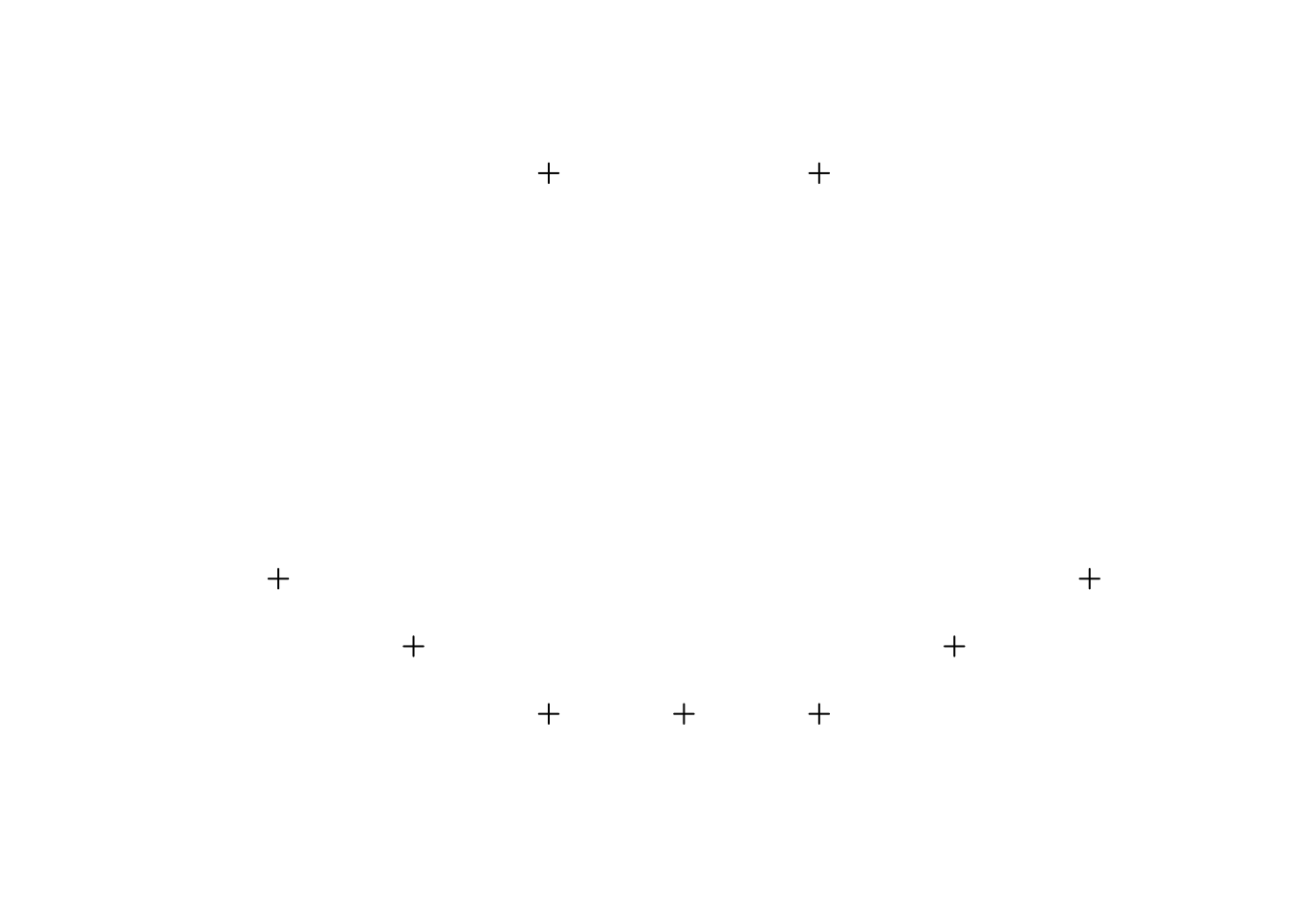To get a summary of how R sees these points, we can ask it for summary information in a couple different ways. Here’s a summary of available commands:

``summary(spatial.points)``
``````## Object of class SpatialPoints
## Coordinates:
##           min max
## coords.x1 0.5 3.5
## coords.x2 0.0 2.0
## Is projected: NA
## proj4string : [NA]
## Number of points: 9``````
``coordinates(spatial.points)``
``````##       coords.x1 coords.x2
##  [1,]       1.5      2.00
##  [2,]       2.5      2.00
##  [3,]       0.5      0.50
##  [4,]       1.0      0.25
##  [5,]       1.5      0.00
##  [6,]       2.0      0.00
##  [7,]       2.5      0.00
##  [8,]       3.0      0.25
##  [9,]       3.5      0.50``````

## Coordinate Reference System (CRS)

A `SpatialPoints` object has the ability to keep track of how the coordinates of its points relate to places in the real world through an associated “Coordinate Reference System” (CRS – the combination of a geographic coordinate system and possibly a projection), which is stored using a code called a `proj4string`.

### Terminology

1. Projection: Among cartographers, the term “projection” is synonymous with Flattening Function. All Flattening Functions are referred to as projections. However, in some programs (like ArcGIS), the term projection (or the term Projected Coordinate System) is used to refer to a bundle that includes both a Globe Model and a Flattening Model.
2. Coordinate Reference System (CRS): CRS is the term used by GIS packages in R to define both a Globe Model and a Flattening Model.
3. Projected Coordinate System: The ArcGIS term for a bundle of both a Globe Model and a Flattening Model
4. Geographic Coordinate System: The ArcGIS term for just a Globe Model. If you specify a Geographic Coordinate System but not a Projected Coordinate System, ArcGIS will often refuse to execute certain tools. However, it will still show you an image of your data, often by just using a Plate Carrée projection.

CRS objects can be created by passing the `CRS()` function the code associated with a known projection. You can find the codes for most commonly used projections from www.spatialreference.org or https://epsg.io/.

The most commonly used CRS is the WGS84 latitude-longitude projection. You can create a WGS84 lat-long projection object by either passing the reference code for the projection – `CRS("+init=EPSG:4326"`) – or by directly calling all its relevant parameters – `CRS("+proj=longlat +ellps=WGS84 +datum=WGS84 +no_defs")`.

``is.projected(spatial.points) # see if a projection is defined. ``
``##  NA``
``````crs.geo <- CRS("+init=epsg:32633")  # looks up UTM 33N
proj4string(spatial.points) <- crs.geo  # define projection system of our data
is.projected(spatial.points)``````
``##  TRUE``
``summary(spatial.points)``
``````## Object of class SpatialPoints
## Coordinates:
##           min max
## coords.x1 0.5 3.5
## coords.x2 0.0 2.0
## Is projected: TRUE
## proj4string :
## [+init=epsg:32633 +proj=utm +zone=33 +datum=WGS84 +units=m +no_defs
## +ellps=WGS84 +towgs84=0,0,0]
## Number of points: 9``````

When `CRS` is called with only an EPSG code, R will try to complete the CRS with the parameters looked up in the EPSG table:

``````crs.geo <- CRS("+init=epsg:32633")  # looks up UTM 33N
crs.geo # prints all parameters``````
``````## CRS arguments:
##  +init=epsg:32633 +proj=utm +zone=33 +datum=WGS84 +units=m +no_defs
## +ellps=WGS84 +towgs84=0,0,0``````

## Data manipulation

Geometry-only objects (objects without attributes) can be subsetted similar to how vectors or lists are subsetted; we can select the first two points by

``spatial.points[1:2]``
``````## class       : SpatialPoints
## features    : 2
## extent      : 1.5, 2.5, 2, 2  (xmin, xmax, ymin, ymax)
## coord. ref. : +init=epsg:32633 +proj=utm +zone=33 +datum=WGS84 +units=m +no_defs +ellps=WGS84 +towgs84=0,0,0``````

Moving from a `SpatialPoints` to a `SpatialPointsDataFrame` occurs when you add a `data.frame` of attributes to the points. Let’s just add an arbitrary table to the data – this will label each point with a letter and a number.

Note points will merge with the `data.frame` based on the order of observations.

``````df <- tibble(attr1 = letters[1:9],  # needs tidyverse library
attr2 = c(101:109))
glimpse(df)``````
``````## Observations: 9
## Variables: 2
## \$ attr1 <chr> "a", "b", "c", "d", "e", "f", "g", "h", "i"
## \$ attr2 <int> 101, 102, 103, 104, 105, 106, 107, 108, 109``````
``````first.spdf <- SpatialPointsDataFrame(spatial.points, df)
summary(first.spdf)``````
``````## Object of class SpatialPointsDataFrame
## Coordinates:
##           min max
## coords.x1 0.5 3.5
## coords.x2 0.0 2.0
## Is projected: TRUE
## proj4string :
## [+init=epsg:32633 +proj=utm +zone=33 +datum=WGS84 +units=m +no_defs
## +ellps=WGS84 +towgs84=0,0,0]
## Number of points: 9
## Data attributes:
##     attr1               attr2
##  Length:9           Min.   :101
##  Class :character   1st Qu.:103
##  Mode  :character   Median :105
##                     Mean   :105
##                     3rd Qu.:107
##                     Max.   :109``````

Now that we have attributes, we can also subset our data the same way we would subset a `data.frame`. Some subsetting:

``first.spdf[1:2, "attr1"] # row 1 and 2 only, attr1``
``````## class       : SpatialPointsDataFrame
## features    : 2
## extent      : 1.5, 2.5, 2, 2  (xmin, xmax, ymin, ymax)
## coord. ref. : +init=epsg:32633 +proj=utm +zone=33 +datum=WGS84 +units=m +no_defs +ellps=WGS84 +towgs84=0,0,0
## variables   : 1
## names       : attr1
## min values  :     a
## max values  :     b``````

### SpatialPoint from a lon/lat table

A `SpatialPointsDataFrame` object can be created directly from a `data.frame` by specifying which columns contain the coordinates.

This is interesting, for example if you have a spreadsheet that contains latitude, longitude and some values. You can read this into a data frame with `read.table`, and then create the object from the data frame in one step by using the `coordinates()` command. That automatically turns the dataframe object into a `SpatialPointsDataFrame`.

### Example

``airports <- read_csv("data/ec-airports.csv", comment = "##")``
``````## Parsed with column specification:
## cols(
##   .default = col_character(),
##   id = col_double(),
##   latitude_deg = col_double(),
##   longitude_deg = col_double(),
##   elevation_ft = col_double(),
##   scheduled_service = col_double(),
##   score = col_double(),
##   last_updated = col_datetime(format = "")
## )``````
``## See spec(...) for full column specifications.``
``````coordinates(airports) <- c("longitude_deg", "latitude_deg")
plot(airports)``````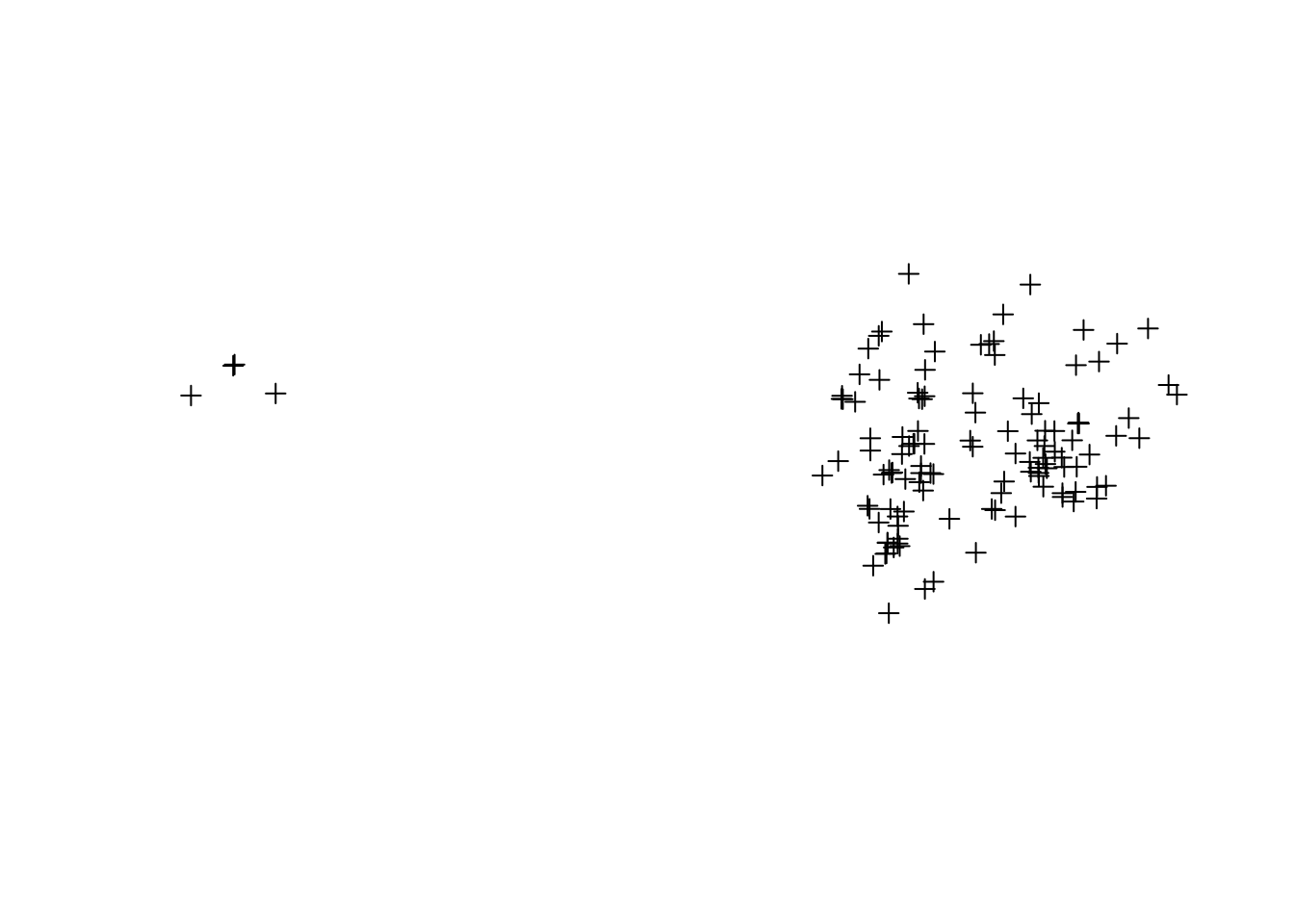``airports <- read_csv("data/ec-airports.csv", comment = "##")``
``````## Parsed with column specification:
## cols(
##   .default = col_character(),
##   id = col_double(),
##   latitude_deg = col_double(),
##   longitude_deg = col_double(),
##   elevation_ft = col_double(),
##   scheduled_service = col_double(),
##   score = col_double(),
##   last_updated = col_datetime(format = "")
## )``````
``## See spec(...) for full column specifications.``
``````coordinates(airports) <- c("longitude_deg", "latitude_deg")
plot(airports)````````````library(leaflet)
popup = ~municipality)``````

### SpatialPolygons

To get a `SpatialPolygons` object, we have to build it up by:

1. creating `Polygon` objects,
2. combining those into `Polygons` objects, and finally
3. combining those to create `SpatialPolygons`.
• A `Polygon` object is a single geometric shape (e.g. a square, rectangle, etc.) defined by a single uninterrupted line around the exterior of the shape.
• A `Polygons` object consists of one or more simple geometric objects (`Polygon` objects) that combine to form what you think of as a single unit of analysis (an “observation”).
• A `SpatialPolygons` object is a collection of `Polygons` objects, where each `Polygons` object is an “observation”.

If you’re familiar with shapefiles, `SpatialPolygons` is basically the R analogue of a `shapefile` or `layer`.

One special note: if you want to put a hole in a polygon (e.g. if you wanted to draw South Africa and leave a hole in the middle for Lesotho) you do so by (a) creating a `Polygon` object for the outline, (b) creating a second `Polygon` object for the hole and passing the argument `hole=TRUE`, and (c) combine the two into a `Polygons` object.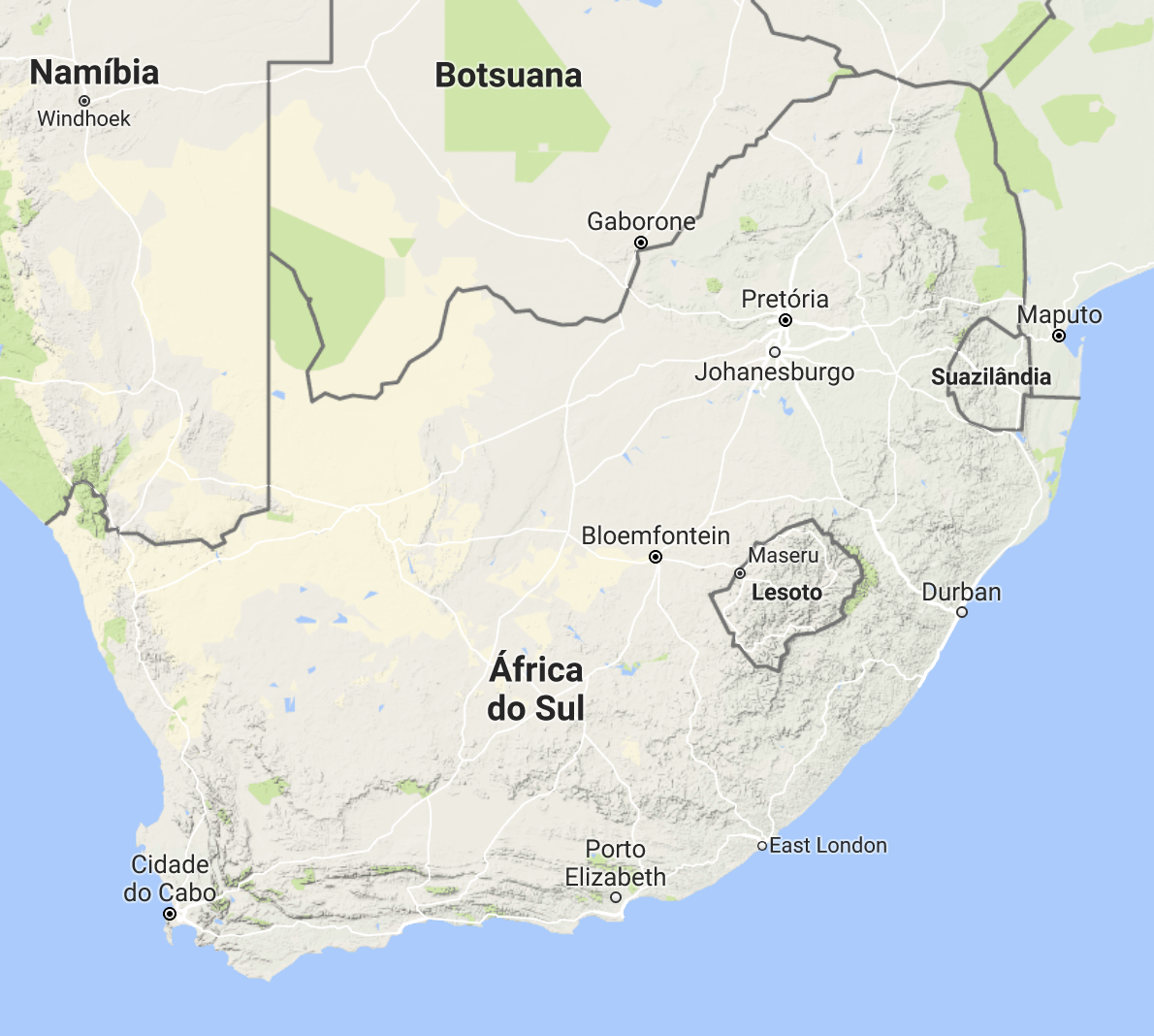South Africa

SpatialPolygons object is a bit harder, but stil relatively straightforward with the `spPolygons` functions (from the `raster` package).

``````crdref <- CRS('+proj=longlat +datum=WGS84')
lon <- c(-116.8, -114.2, -112.9, -111.9, -114.2, -115.4, -117.7)
lat <- c(41.3, 42.9, 42.4, 39.8, 37.6, 38.3, 37.6)
lonlat <- cbind(lon, lat)
pols <- spPolygons(lonlat, crs=crdref)
pols``````
``````## class       : SpatialPolygons
## features    : 1
## extent      : -117.7, -111.9, 37.6, 42.9  (xmin, xmax, ymin, ymax)
## coord. ref. : +proj=longlat +datum=WGS84 +ellps=WGS84 +towgs84=0,0,0``````

We can make use generic R function plot to make a map.

``````pts <- SpatialPoints(lonlat)
plot(pols, axes=TRUE, las=1)
plot(pols, border='blue',
col='yellow', lwd=3,
points(pts, col='red',
pch=20, cex=3)``````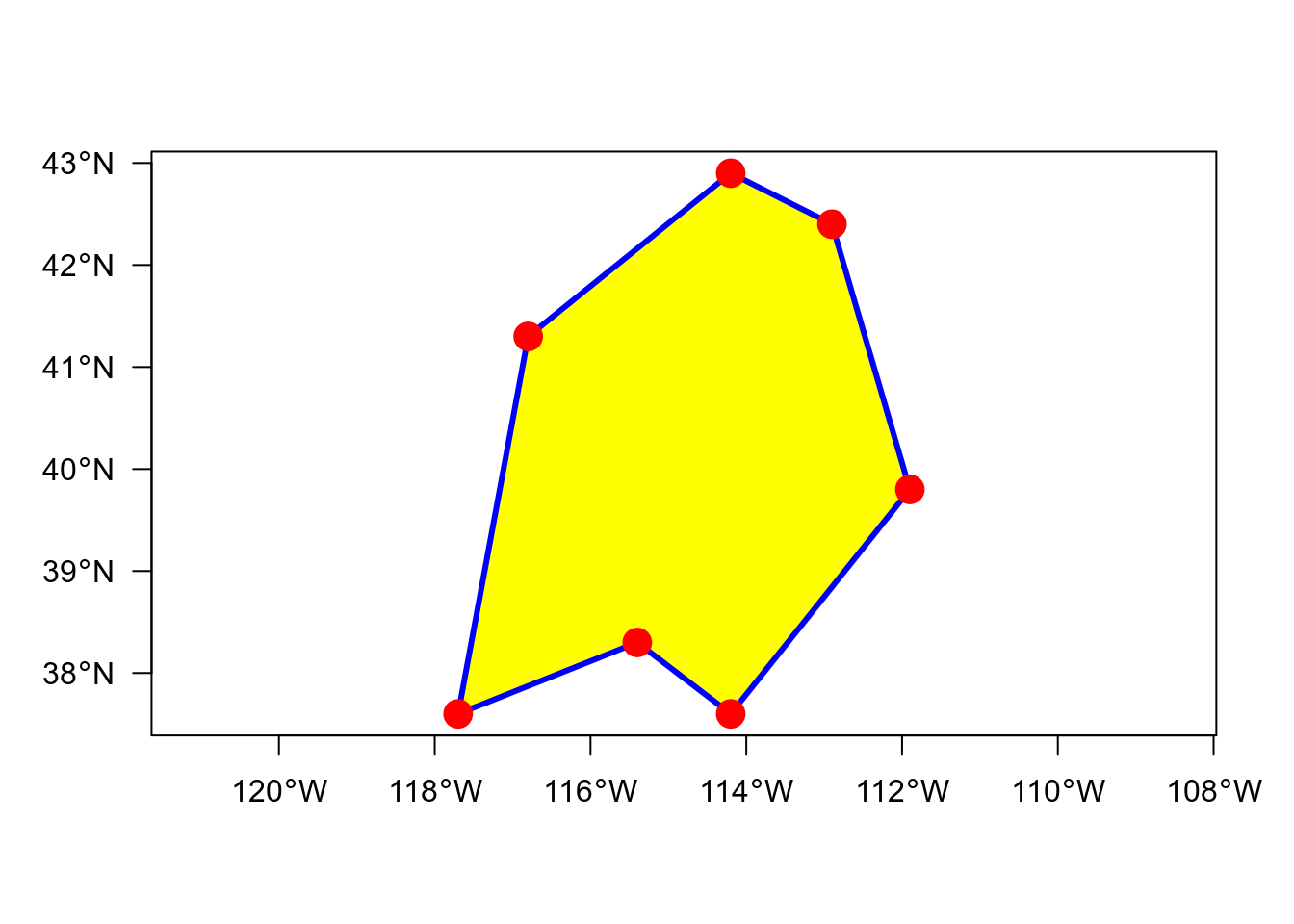``````leaflet() %>% addTiles() %>% addCircleMarkers(data = pts, color = "red") %>%
addPolygons(data = pols, color = "blue", fillColor = "yellow")``````
``````# create polyon objects from coordinates
Sr1 <- Polygon(cbind(c(2, 4, 4, 1, 2), c(2, 3, 5, 4, 2)))
Sr2 <- Polygon(cbind(c(5, 4, 2, 5), c(2, 3, 2, 2)))
Sr3 <- Polygon(cbind(c(4, 4, 5, 10, 4), c(5, 3, 2, 5, 5)))
Sr4 <- Polygon(cbind(c(5, 6, 6, 5, 5), c(4, 4, 3, 3, 4)), hole = TRUE)

# create lists of polygon objects from polygon objects and unique ID
Srs1 <- Polygons(list(Sr1), "s1")
Srs2 <- Polygons(list(Sr2), "s2")
Srs3 <- Polygons(list(Sr3, Sr4), "s3/4")

# create spatial polygons object from lists
SpP <- SpatialPolygons(list(Srs1, Srs2, Srs3), 1:3)``````
``plot(SpP)``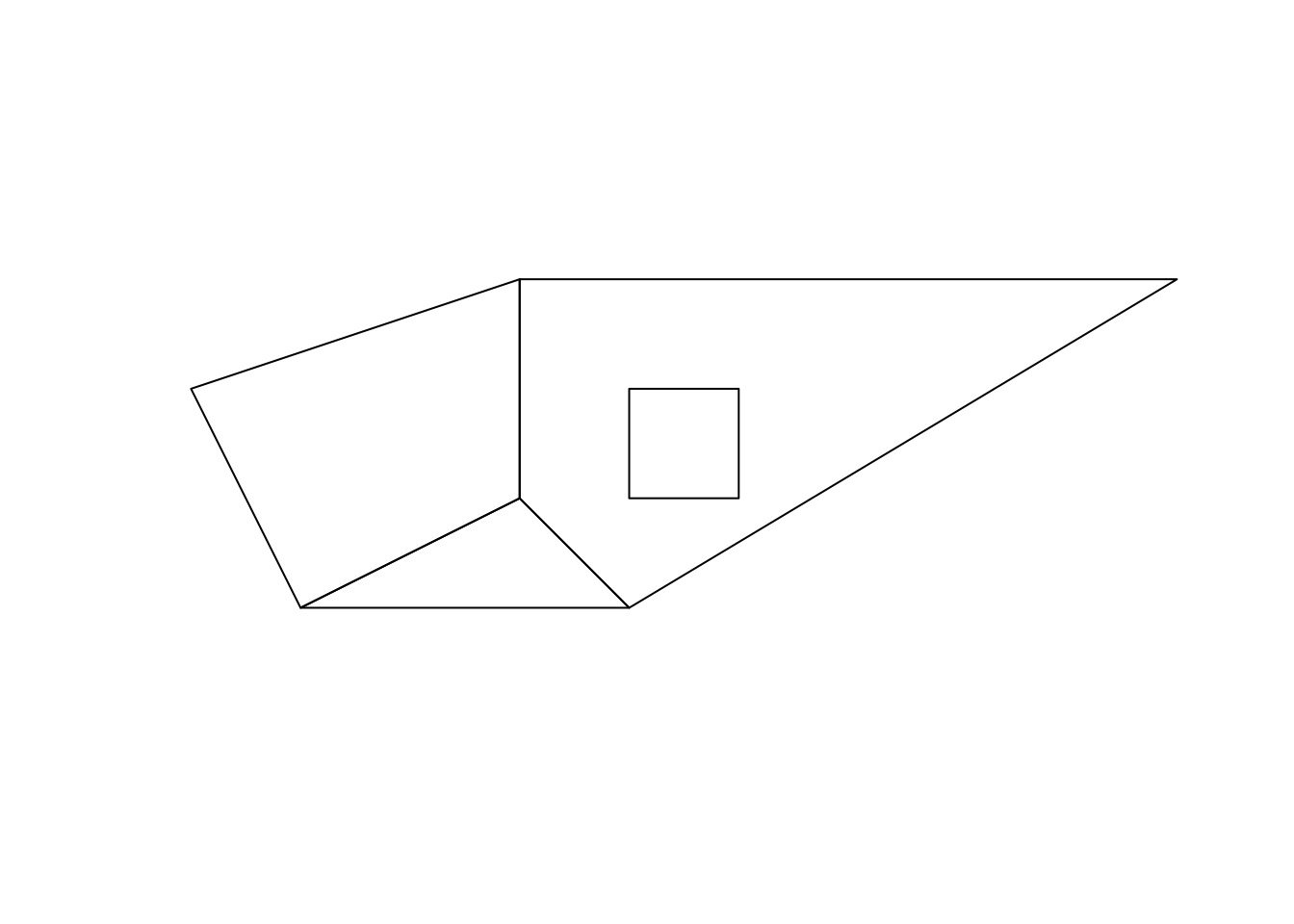### SpatialPolygonsDataFrame

``````attr <- data.frame(numbers = 1:3,
characters = LETTERS[3:1],
row.names = c("s3/4", "s1", "s2"))
SpDf <- SpatialPolygonsDataFrame(SpP, attr)``````
``spplot(SpDf)``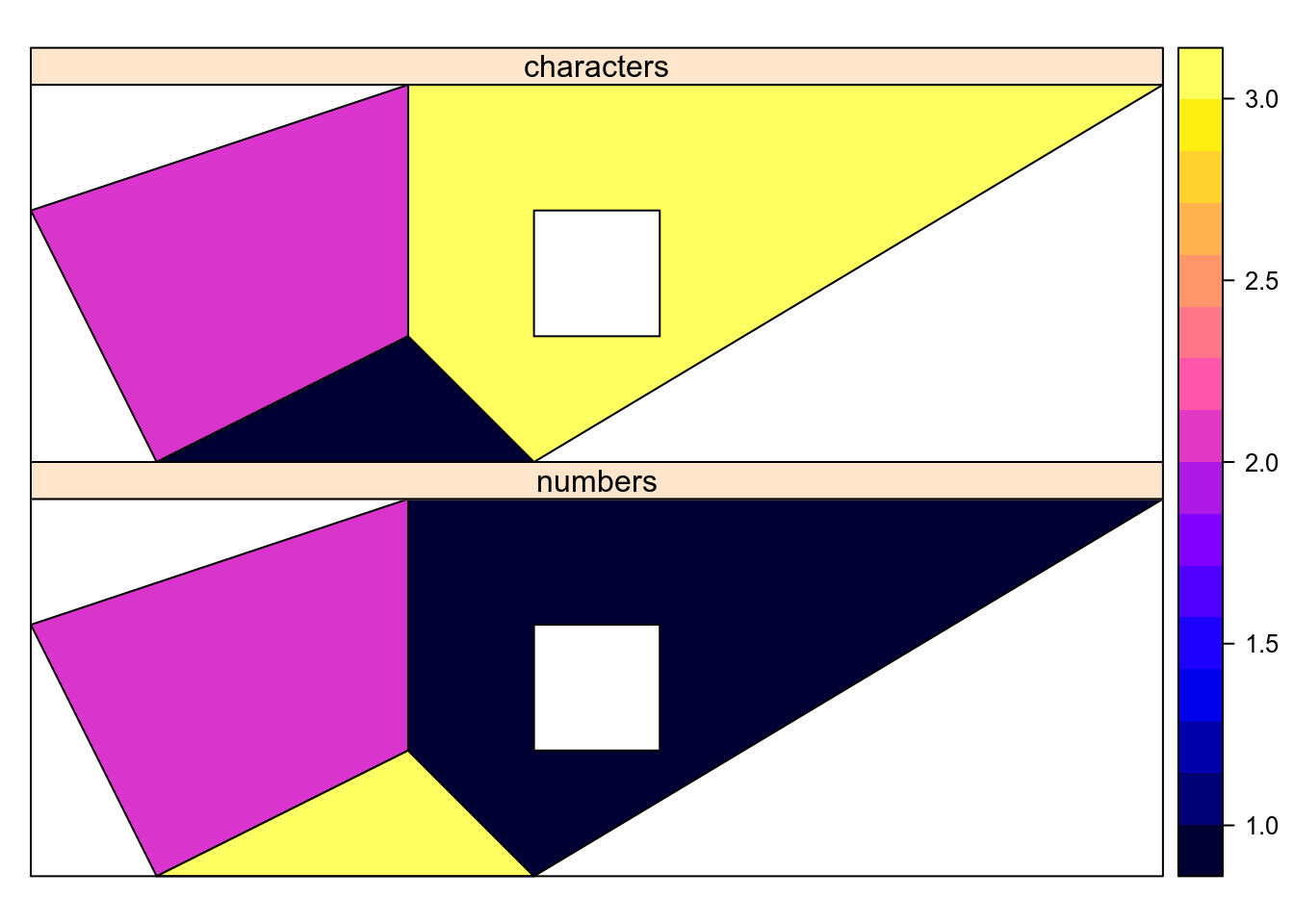`SpatialLines` objects are basically like `SpatialPolygons`, except they’re built up using Line objects (each a single continuous line, like each branch of a river), Lines objects (collection of lines that form a single unit of analysis, like all the parts of a river), to `SpatialLines` (collection of “observations”, like rivers).

## Data acquisition

### SRTM, Worldclim, Global adm. boundaries

The raster package is a great tool for raster processing and calculation but also very useful for data acquisition. With the function `getData()` you can download the following data directly into R:

• SRTM 90 — The Shuttle Radar Topography Mission (elevation data with 90m resolution between latitude -60 and 60).
• World Climate Data (Tmin, Tmax, Precip, BioClim)
• Global adm. boundaries (different levels)

``````library(raster)
``````## Formal class 'SpatialPolygonsDataFrame' [package "sp"] with 5 slots
##   ..@ data       :'data.frame':  1 obs. of  2 variables:
##   .. ..\$ GID_0 : chr "ECU"
##   .. ..\$ NAME_0: chr "Ecuador"
##   ..@ polygons   :List of 1
##   .. ..\$ :Formal class 'Polygons' [package "sp"] with 5 slots
##   ..@ plotOrder  : int 1
##   ..@ bbox       : num [1:2, 1:2] -92.01 -5.02 -75.19 1.68
##   .. ..- attr(*, "dimnames")=List of 2
##   ..@ proj4string:Formal class 'CRS' [package "sp"] with 1 slot``````

``````ecuador_level0 %>% leaflet() %>% addTiles() %>%